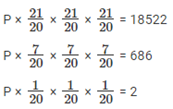# A person invested a certain amount of money - Quantitative Aptitude - Interest

## CAT 2020 - Slot 3 - Quantitative Aptitude - Interest - Question 20 - A person invested a certain amount of money

Q. 1: 20. A person invested a certain amount of money at 10% annual interest, compounded half-yearly. After one and a half years, the interest and principal together became Rs 18522. The amount, in rupees, that the person had invested is

A 10% annual interest is a 5% bi-annual interest.

Hence interst for half an year is 5%.

The Principal, P is invested for 1.5 years or 3 terms of half years.

Therefore, P (1 + 5%)3 = 18522

P(1.05)3 = 18522P = 2 × 20 × 20 × 20 = 16000.

### Past Year Question Paper & SolutionsCounselling Session
By IIM Mentor

#### Free Material Area